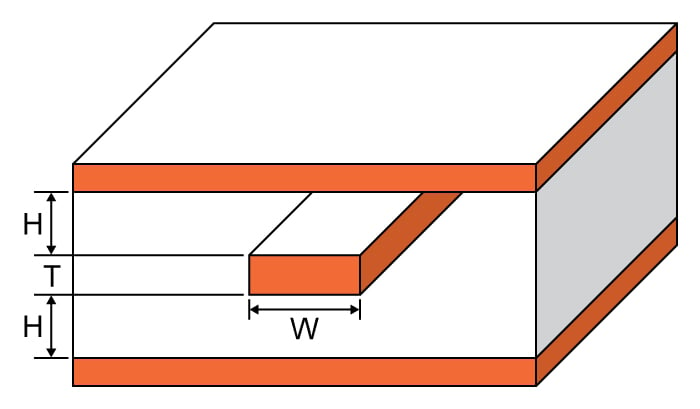# Symmetric Stripline Impedance Calculator

## This calculator helps you compute the characteristic impedance of a symmetric stripline.

Ohms

### Overview

This calculator is designed to calculate the characteristic impedance of a symmetric stripline - a flat conductor between two ground planes whose distance between the conductor and the planes is the same for both reference planes.

Just enter the given values for trace thickness, substrate heights, trace width, and substrate dielectric in the calculator above and press the "calculate" button. The default units for all given values, except the substrate dielectric, is in mil. It is possible to select other units.### Equations

When $$\frac{W}{b}<.35$$ or $$\frac{T}{b}≤.25$$ or $$\frac{t}{W}≤.11$$:

$$Z_{0_{ss}}=\frac{\eta _{0}}{2\pi \sqrt{er}}ln\left [ 1+\frac{8h}{\pi W_{eff}} \left ( \frac{16h}{\pi W_{eff}} \right )+\sqrt{(\frac{16h}{\pi W_{eff}})^2+6.27}\right ]$$

Else,

$$Z_{0_{ss}}=\frac{94.15}{\left [ \frac{\frac{W}{b}}{1-\frac{T}{b}}+\frac{\theta }{\pi} \right ]}$$

Where:

$$W_{eff}=W+\frac{T}{\pi}ln\left [ \frac{e}{\sqrt{(\frac{T}{4H+T})^2+(\frac{\pi T}{4(W+1.1T)})^m}} \right ]$$

$$m = \frac{6h}{3h+t}$$

$$b = 2h+t$$

$$Z_{0_{ss}}$$ = characteristic impedance of the symmetric stripline in ohms (Ω).

$$H_{1}$$ = subtrate height 1

$$H_{2}$$ = subtrate height 2

$$W$$ = trace width

$$T$$ = trace thickness

$$\epsilon_{r}$$ = substrate dielectric

### Applications

The stripline has the advantage over the microstrip to improve isolation between adjacent traces. A case where an engineer would use a symmetrical stripline would be when signals need to evenly propagate to the reference planes. For example, a transmission needs to route to an internal layer and is coming onto a board through a coaxial cable. If it is required to maintain the balance between reference planes, a symmetrical construction would be needed. Symmetrical striplines also avoid inductance and capacitance across planes and signals as compared to a coplanar setup.